International
Tables for
Crystallography
Volume D
Physical properties of crystals
Edited by A. Authier

International Tables for Crystallography (2006). Vol. D, ch. 1.1, p. 13

## Section 1.1.4.5.3.1. Tensors of rank 2

A. Authiera*

aInstitut de Minéralogie et de la Physique des Milieux Condensés, Bâtiment 7, 140 rue de Lourmel, 75015 Paris, France
A bilinear form is said to be antisymmetric if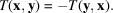Its components satisfy the relations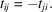The associated matrix, T, is therefore also antisymmetric: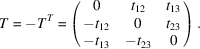The number of independent components is equal to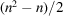, where n is the number of dimensions of the space. It is equal to 3 in a three-dimensional space, and one can consider these components as those of a pseudovector or axial vector. It must never be forgotten that under a change of basis the components of an axial vector transform like those of a tensor of rank 2.
Every tensor can be decomposed into the sum of two tensors, one symmetric and the other one antisymmetric: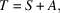with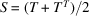and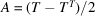.
Example. As shown in Section 1.1.3.7.2, the components of the vector product of two vectors, x and y,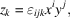are really the independent components of an antisymmetric tensor of rank 2. The magnetic quantities, B, H (Section 1.1.4.3.2), the tensor representing the pyromagnetic effect (Section 1.1.1.3) etc. are axial tensors.